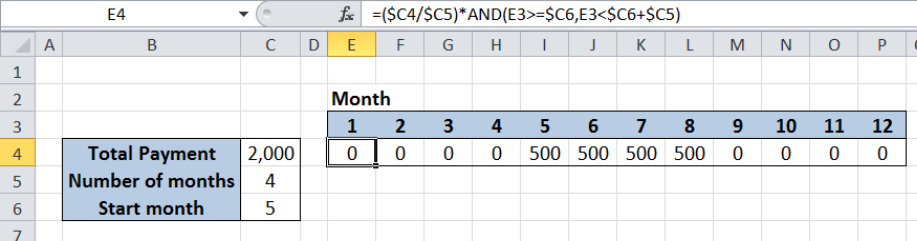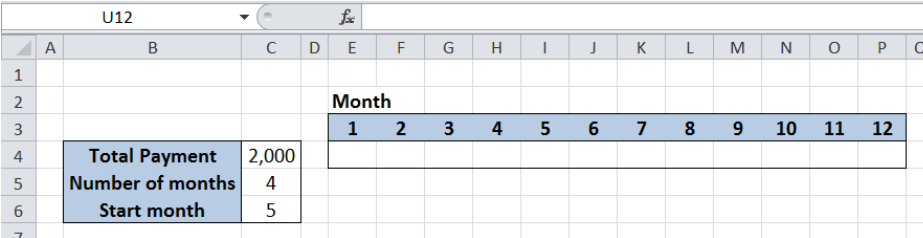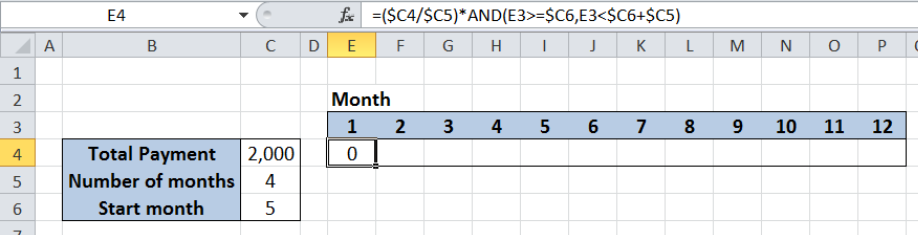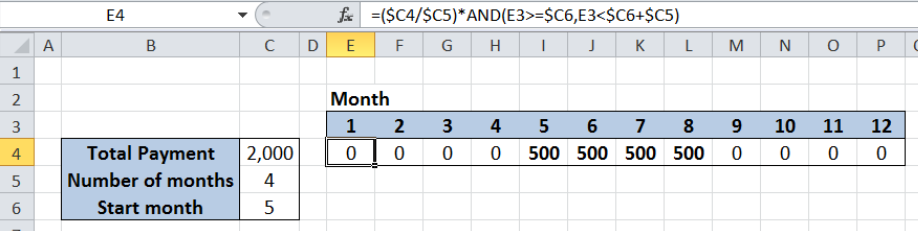Get instant live expert help with Excel or Google Sheets“My Excelchat expert helped me in less than 20 minutes, saving me what would have been 5 hours of work!”

#### Post your problem and you’ll get Expert help in seconds.

Your message must be at least 40 characters
Our professional Expert are available now. Your privacy is guaranteed.

# Split payment across months

While working with Excel, we are able to distribute a certain amount across a period of time based on some given conditions by using the AND function.  This step by step tutorial will assist all levels of Excel users in splitting payment across months.Figure 1. Final result:  Split payment across months

Final formula: `=(\$C4/\$C5)*AND(E3>=\$C6,E3<\$C6+\$C5)`

## Syntax of AND Function

AND function evaluates all logical tests and returns a TRUE if all arguments are TRUE; FALSE if one or more arguments is FALSE

`=AND(logical1, [logical2], ...)`

The parameters are:

• logical1–  the first condition that we want to test
• only logical1 is required; succeeding conditions are optional

## Setting up our Data

Our data consists of three cells containing the details of the payment.  The total payment is \$2,000 in cell C4. We want to split the payment in 4 months (cell C5) starting from the fifth month (cell C6).  A second table is prepared in cells E3:P4.

The corresponding splitted payment per month will be recorded in cells E4:P4.Figure 2. Sample data to split payment across months

## Split payment across four months

In order to split payment across four months starting from the fifth month, we will use the AND function and some logical tests.  Let us follow these steps:

Step 1.  Select cell E4

Step 2.  Enter the formula: `=(\$C4/\$C5)*AND(E3>=\$C6,E3<\$C6+\$C5)`

Step 3.  Press ENTER

Step 4:  Copy the formula in cell E4 to cells F4:P4 by clicking the “+” icon at the bottom right-corner of cell E4 and dragging it to the right

The dollar symbols “\$” in the formula fix the cells so that we can easily copy and paste the formula to other cells.Figure 3. Entering the formula using AND function

Our formula has two parts. The first part \$C4/\$C5 simply divides total payment by the number of months, in order to determine the splitted payment per month.  The second part `AND(E3>=\$C6,E3<\$C6+\$C5``)`  determines which months should the payment be made.  The start month is cell C6 while the end month is the sum of the start month (C6) and the number of months (C5).

The AND function returns TRUE if both conditions are satisfied:

• Greater than or equal to start month
• Less than the end month

As a result, our formula returns a zero “0” value for FALSE, and returns the splitted payment for TRUE.  As shown below, the splitted payment of 500 is recorded in four months, starting from the fifth month up to the eighth month.Figure 4. Output: Split payment across four months starting from 5th month

Most of the time, the problem you will need to solve will be more complex than a simple application of a formula or function. If you want to save hours of research and frustration, try our live Excelchat service! Our Excel Experts are available 24/7 to answer any Excel question you may have. We guarantee a connection within 30 seconds and a customized solution within 20 minutes.

### Did this post not answer your question? Get a solution from connecting with the expert.Another blog reader asked this question today on Excelchat:
Related blogs
Solution examplesYes hello Im looking for help on conditional formatting I currently have a conditional format for D9>D32 and so on from D9:S20. I need to add an if statement that references cell D4 if the first four characters are 1358 for the rule to apply can you please assist?
Solved by Z. U. in 20 minsUse the Vlookup Function to complete the "employee" column of table 2. Use "job Id" from table 2 as your lookup_value(s) and table 1 as your reference.
Solved by C. H. in 16 minsIf a cell in another sheet is populated I need a vlookup done. If the cell is not populated I need the cell to return blank.
Solved by T. D. in 60 minsI am trying to make a chart that turns a week range red if nothing is entered in the range. If something is entered then I would like it to turn green. Please Help
Solved by E. U. in 43 minsI need a check box to show/hide an answer of an if function
Solved by Z. U. in 23 mins## Subscribe to Excelchat.coAnother blog reader asked this question today on Excelchat: